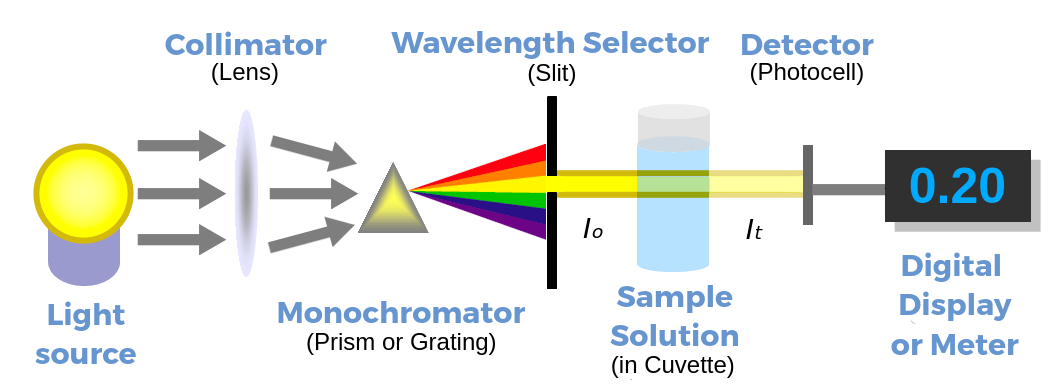A spectrometer is an instrument that provides information about the intensity of radiated energy. A spectrophotometer is an instrument that determines the ratio between the intensity of light emitted from an internal source and that which passes through a given solution. This ratio can be used to determine the concentration of dissolved molecules in a sample.

Before a sample is measured, the spectrophotometer is set to measure at a certain wavelength. The detected wavelength can be tuned to a value optimal for measuring a specific compound.

On measurement, the spectrophotometer returns data as absorbance values (A). Absorbance is calculated as log(I0/It), where I0 is the intensity of the incident light (light falling onto a sample) and It is the intensity of the light that passes through the solution and onto a photovoltaic detector.

The relationship between absorbance and concentration is linear and is described by Beer-Lambert Law:

A = εcl

where c is the concentration of the solution, l is the pathlength, otherwise described as the distance traveled by light when passing through a solution (typically the width of a cuvette), and ε is the extinction coefficient, which is specific for a compound.Figure 1: Components of a spectrophotometer: Light emitted from the source passes through the slit, letting only 1 specific wavelength through. This light partially passes through the sample placed in a cuvette and the detector measures the intensity on the other side of the cuvette.

In an example, we will measure concentrations of NADH. NADH has an absorption maximum at 340 nm; therefore, the spectrophotometer is set to measure at this wavelength. The extinction coefficient for NADH, ε = 6220 M-1cm-1. The pathlength is the width of the cuvette, which is 1 cm.

There are certain limitations of the Beer-Lambert Law that spectrophotometer users must consider. Some of these are related to technical issues; however, the law does have a real limitation; because it only applies to dilute solutions. When the concentration of an absorbing species increases, so does the frequency of physicochemical interactions among the molecules. Thus, at a given concentration, the molecules will begin to affect the charge distribution of the neighbor molecules. When this occurs, the relationship between absorbance and concentration is no longer linear. As a rule of thumb, one should stay below an absorption value of 1 when doing measurements.

Absorbance is inversely proportional to transmittance (light passing through a sample that is not absorbed). When absorbance = 1, 10% of the light is transmitted through the sample, at 2, 1% of the light is transmitted, and so on in a logarithmic trend.